Next: 6.3 Simulation of GAIA Up: 6.2 GAIA BBP vs Previous: 6.2.2 Point Spread Function   Contents

## 6.2.3 Noise

Any astronomical observation is affected by different noise'' sources. These can be roughly divided into natural and artificial sources. Natural sources essentially are the signal noise connected with the quantum nature of light, to the background noise due to the radiation coming from natural sources different from the observed object, and including in particular the sky background and the cosmic ray hits. Artificial sources include the dark noise that a detector may generate even in absence of any signal, the readnoise originating from the reading process and the non-optimal CCD performance over the focal plane.

For the purpose of our simulations, most of these sources can be neglected. For instance, due to GAIA short one-scan exposure time, the rate of cosmic ray hits will be very low, and moreover the superposition of many scans of the same sky region will average over these unlikely events. Likewise, the superposition of different scans will average over possible space or time variations in the CCD response. The effects of the sky background and of the CCD dark current will be order of magnitudes lower than the signal noise and the readnoise. On the whole, the signal noise and readnoise appear to dominate the noise budget. Accordingly, only signal noise and readnoise heve been considered in the simulations.

The signal noise is usually assumed to follow a Poisson distribution and the relative standard error in surface photometry is thus proportional to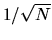, where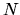is the total number of detected electrons per unit area. Since the exposure time of the WFPC2 images used in the simulations is much bigger than the one-scan GAIA exposure time, and since in Subsection 6.2.1 the electron count rate of the two instruments was found to be almost the same, it is concluded that the total number of detected electrons per unit area is much bigger in WFPC2 images than in GAIA observations, and thus that the signal noise present in WFPC2 images is negligible with respect to that introduced by GAIA.

On the other hand, the readnoise is usually assumed to follow a Gaussian distribution with zero mean and standard deviation independent from the total number of detected electrons per unit area. Accordingly, the relative standard error in surface photometry is proportional to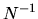. Measurements of HST WFPC2 readnoise yielded average values of about 5 e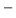/pixel rms and 7 e/pixel rms, depending on the chosen analog-to-digital conversion gain (see [Biretta et al. 1996]). The readnoise levels expected in GAIA Astro CCDs for different choices of the sample size and full CCD readout was discussed in Section 5.5, and results were given in Table 5.2. The readnoise levels per pixel appear to be similar in the two cases. As discussed with reference to the signal noise, however, the important figure in comparing the two instruments' performance is the relative standard error, and for the same reasons discussed above one can conclude that the readnoise present in WFPC2 images is negligible with respect to that introduced by GAIA.

Note that all readnoise values estimated for GAIA BBP and reported in Table 5.2 were calculated assuming full CCD readout. Obviously, if this requirement is relaxed, a smaller readnoise would yield an higher accuracy in surface photometry, while still allowing to fully image most detected galaxies. For instance, according to Table 4.4, the effective radius of a typical galaxy of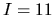is about 25 arcsec, meaning that under the assumption of circular symmetry the circle enclosing half its light is well within a diameter of 2 arcmin. The across-scan size of a BBP CCD is about 4 arcmin, so that for a galaxy of this magnitude only half the CCD may be readout, thus giving a lower reading frequency and therefore a lower readnoise than that expected for full CCD readout with the same sample size. Alternatively, the sample size could be reduced giving equal reading frequency but smaller sample size, and thus higher angular resolution. Since only some 2000 galaxies out of the 3 million we expect to observe are brighter than, most of the time we could even readout the CCD more slowly than suggested above in order to further reduce readnoise or increase angular resolution. In so doing, during a single scan we would be able to observe only some parts (e.g. those near the center of each CCD) of brighter galaxies, which could lead to a low number of total scans for certain sky regions and as a consequence to problems in their stacking, but this could be acceptable in view of an higher accuracy in surface photometry or an higher angular resolution. Although very interesting, this possibility is however not considered in the following.Next: 6.3 Simulation of GAIA Up: 6.2 GAIA BBP vs Previous: 6.2.2 Point Spread Function   Contents
Mattia Vaccari 2000-12-05